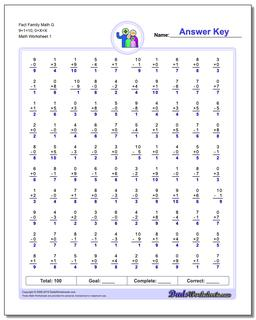# Math Worksheets: Fact Family Math: Fact Family Math: Fact Family Math G 9+1=10, 0+X=X## Fact Family Math G 9+1=10, 0+X=X

PropertyValue
DescriptionFact Family Math G 9+1=10, 0+X=X: These math worksheets have 100 addition and subtraction fact family problems and make for a challenging two minute test.
Resource TypeWorksheet